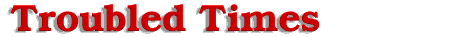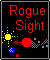#Orbit

To complete all calculations, it must take into account the Earth's orbital position as it revolves around the Sun when the 12th planet passes it. The unknown distance from the 12th planet's position as it passes the Sun to Earth orbit intercept, is needed to find the time interval the 12th planet takes to move from Sun passage to Earth passage. This time frame will give us a start date for the distance table. Although the mechanics of the outgoing orbit is different than the incoming phase of the orbit, before passage of the Sun, we can still use the exponential function to explain the movement of the 12th planet out of in the inner part of the solar system. Due to the premise, that most of the orbital variables, the repulsion and gravitational forces emanating from the Sun, the planets, and too a lesser degree the Dark One effecting the 12th planet's path as it moves around the backside of the Sun, occur after Earth passage. To solve the equation we will use what information that is given to us. We are told that the 12th planet traverses Saturn's orbital diameter in 3 months and passes the Sun between the orbits of Mercury and Venus. The length of the orbital track on the far side of the Sun to its furthest point in the elliptical orbit is 3.56 S-P units.

To solve for how the 12th planet moves after it passes the Sun, there are several steps necessary. The 12th planet moves from an incoming to an outgoing solar object as it passes the Sun. The (k) rate, representing incoming acceleration in the exponential equation changes a from a positive value when the 12th planet hurtles towards the Sun, to a negative value as the combined forces of gravity from both binary stars erodes the 12th planet's forward momentum. Step one will be to find the incoming transit time of the 12th planet from the orbit of Saturn to the Sun. Using the same formulas derived previously in the text, you can solve for incoming time (t). Distance(final) equals 9.362 S-P units, Distance(initial) equals 9.362 - .241496588 (distance to Saturn) or 9.1205 S-P units and (k) incoming equals .03232169 giving us an incoming transit time of the 12th planet from Saturn to the Sun at 0.808557 weeks.

The equation proceeds as follows:

Distance(final) = Distance(initial) e ^ [ (k) *(t)]
LN [Distance(final) / Distance(initial)] = k * t
LN [ 9.362 / 9.1205] / k = t
LN [1.0264788] / 0.03232169 = t
0.02613431 / 0.03232169 = t
0.808557 weeks = t
incoming transit time from Saturn to the Sun.

Step 2 is to find the outgoing transit time(t) from the Sun to Saturn by subtracting the incoming time(t) from the total time of transit of 3 months. Total time (t), the total time to transverse orbit is equal to 3 months or 13 weeks. Subtract incoming time (t) .808557 weeks time interval from Saturn to the Sun, and 12.19144256 weeks is equal to outgoing time(t).

The equation proceeds as follows:

Total time - Incoming time = Outgoing time
13 - 0.808557 = 12.19144256 weeks
outgoing time(t) from the Sun to Saturn's orbit.

Step 3 is to find (k) rate for an outgoing 12th planet, using outgoing time(t), the reference points of the Saturn radii and the farthest point of the elliptical orbit on the far side of the Sun, 3.56 units. Solving for the new (k) rate outgoing, Distance(final) [3.56 units minus Saturn's radius] and Distance(initial) the total distance is respectively [3.56 - 0.241496599] or 3.318503401 and 3.56 S-P units. Now we can solve for the new (k) outgoing. Substituting (t) outgoing 12.19144256 weeks into the equation, we find (k) is equivalent to -.005761.

The equation proceeds as follows:

Distance(final) = Distance(initial) * e ^ [ (k) * (t) ]
LN [Distance(final) / Distance(initial)] = (k) * (t)
LN [Distance(final) / Distance(initial)] / t = k
LN [ 3.318503401 / 3.56 ] / 12.19144256 = k
LN [0.932163877] / 12.19144256 = k
-0.0702446646 / 12.19144256 = k
-0.005761 =k r
ate for deceleration of the 12th planet outgoing

Offered by Robert.